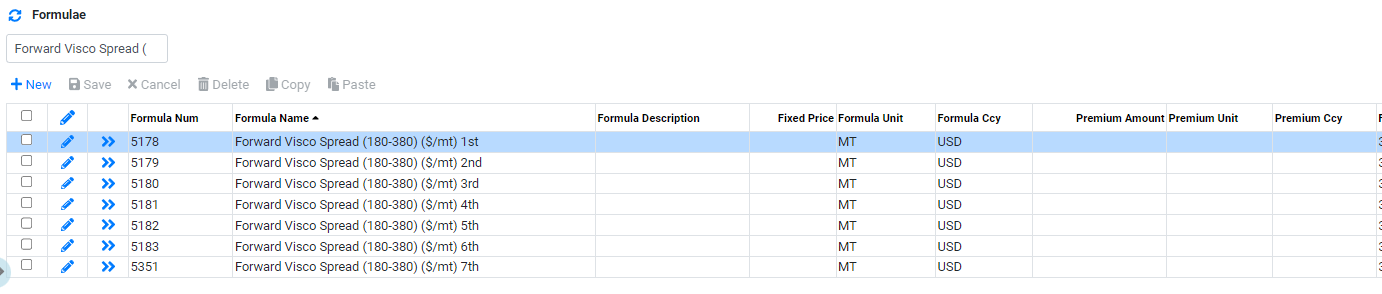Support : User Guide
##### Price Data : Formulae

To add a new formula go to Price Data and then to the Formulae tab.

In CoreTRM, formula can be used to create price that are cacluated using two or more price series. Formula can be used to calculate prices for combination of spot price series or forward price series. It can also be used to add fixed premium/discount to a price series. For example : formula can be used to calculate spread or crack prices based on 2 price series in the system.

`Setting up Formula with Spot Price Series`
`Setting up Formula with Forward Price Series`
###### Setting up Formula with Spot Price Series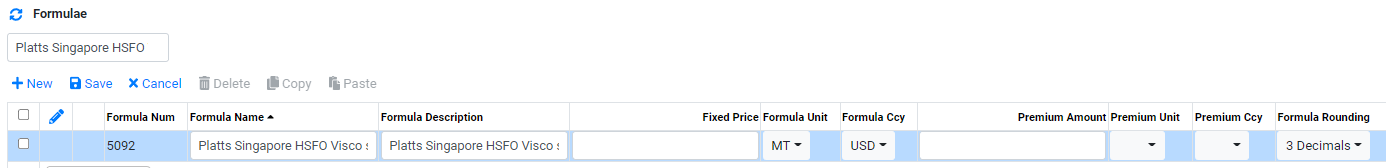To add a formula using spot price series, in formula view:

1. Click on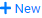to create a new line.

2. In Formula Name, enter the formula name. For example : Platts Singapore HSFO Visco spread (180-380)

3. In Formula Description, enter applicable description for the formula.

4. In Formula Unit, select the unit to be used for this formula.

5. In Formula Ccy, select the currency to be used for this formula.

6. In Premium Amount, enter a premium amount for the formula if a premium or discount is to be applied to all the Formula Item in the formula. For discount, negative sign is to be used before the amount. For example : -2.00.

7. In Premium Unit, select the unit to be used for the premium amount in this formula.

8. In Premium Ccy, select the currency to be used for the premium amount in this formula.

9. In Formula Rounding, select the rounding to be applied for this formula.

10. Clickto confirm creation.

11. To edit formula details after creation, Click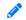to edit.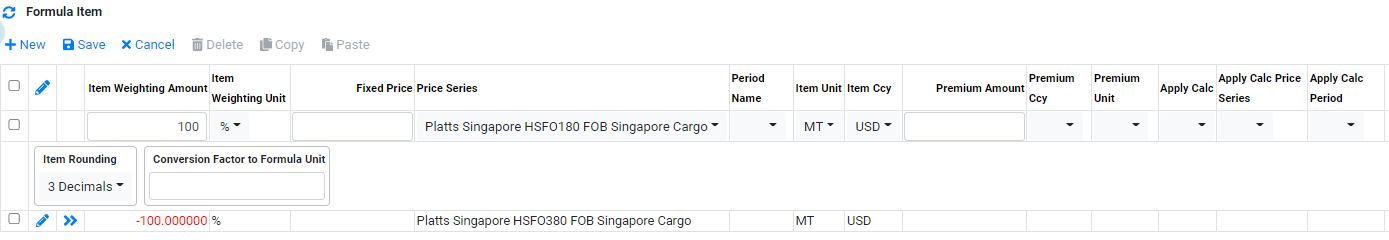To add a formula using spot price series, in formula item view:

1. Click onto create a new line.

2. In Item Weighting Amount, enter the weighting for this item line. For subracting one item line with another, a negaive sign is to be used.

For example, for Visco spread (180-380), the formula is HSFO 180 minus HSFO 380. Therefore the item weighting will be 100% for each line item.

3. In Item Weighting Unit, select the item Weighting Unit. For example, % will be used to calcuate diferences between two prices series or 50% for each price series.

4. In Fixed Price, if a fixed price is to be used as part of the formula calculation.

5. In Price Series, select the spot price series to be used in the formula.

6. In Period Name, select the applicable period for this item line. This is typically used when forward/future price series are used in the formula. For spot price series, Period Name is not required.

7. In Item Unit, select the unit to be used for this item in the formula.

8. In Item Ccy, select the currency to be used for this item in the formula.

9. In Premium Amount, enter a premium amount for the item in the formula if a premium or discount is to be applied to this item in the formula. For discount, negative sign is to be used before the amount. For example : -2.00.

10. In Premium Unit, select the unit to be used for the premium amount used in this formula item.

11. In Premium Ccy, select the currency to be used for the premium amount used in this formula item..

12. In Apply Calc, if the premium/discount is to be multiply by a price series in the formula item, *Price is to be used.

13. In Apply Calc Price Series, it is associated with "Apply Cal". Select the price series to be used in the multiplication.

14. In Apply Calc Period,, select a period is to be used for price series used in the "Apply Cal Price Series". This is typically used for forward price series.

15. Clickto confirm creation.

16. To edit formula item details after creation, Clickto edit.

For example, for a Visco Spread, the formula item will be as per below.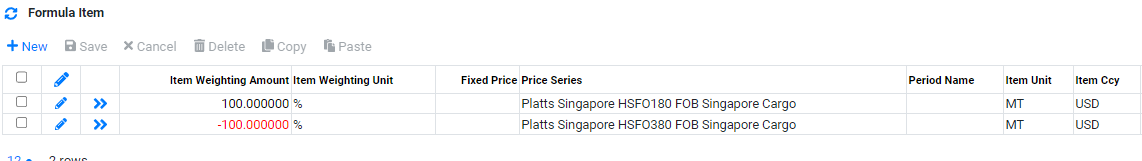###### Setting up Formula with Forward Price Series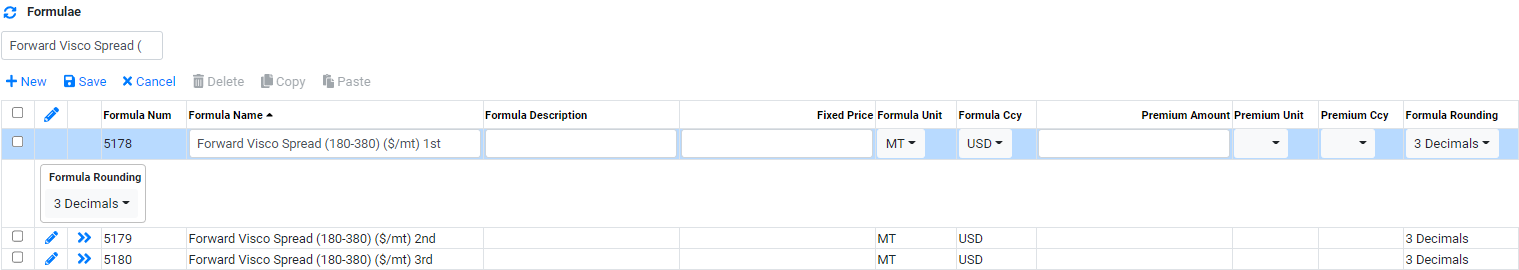To add a formula using spot price series, in formula view:

1. Click onto create a new line.

2. In Formula Name, enter the formula name. For example : Platts Singapore HSFO Visco spread (180-380)

3. In Formula Description, enter applicable description for the formula.

4. In Formula Unit, select the unit to be used for this formula.

5. In Formula Ccy, select the currency to be used for this formula.

6. In Premium Amount, enter a premium amount for the formula if a premium or discount is to be applied to all the Formula Item in the formula. For discount, negative sign is to be used before the amount. For example : -2.00.

7. In Premium Unit, select the unit to be used for the premium amount in this formula.

8. In Premium Ccy, select the currency to be used for the premium amount in this formula.

9. In Formula Rounding, select the rounding to be applied for this formula.

10. Clickto confirm creation.

11. To edit formula details after creation, Clickto edit.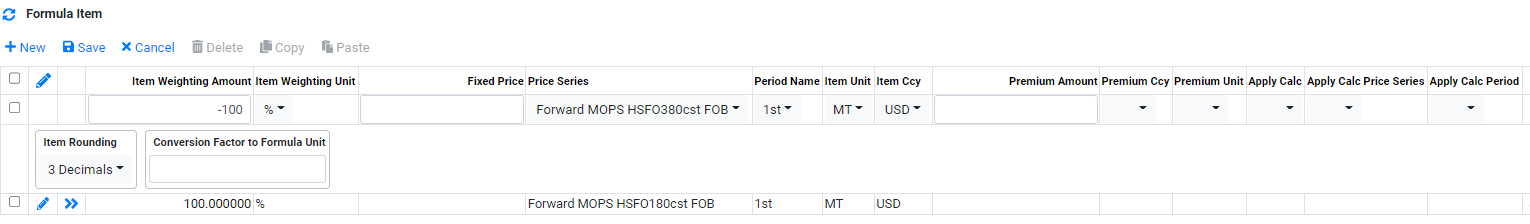To add a formula using forward price series, in formula item view:

1. Click onto create a new line.

2. In Item Weighting Amount, enter the weighting for this item line. For subracting one item line with another, a negaive sign is to be used.

For example, for Visco spread (180-380), the formula is HSFO 180 minus HSFO 380. Therefore the item weighting will be 100% for each line item.

3. In Item Weighting Unit, select the item Weighting Unit. For example, % will be used to calcuate diferences between two prices series or 50% for each price series.

4. In Fixed Price, if a fixed price is to be used as part of the formula calculation.

5. In Price Series, select the foward price series to be used in the formula.

6. In Period Name, select the applicable period for this item line. Typically for formula involving forward price series, for every 1 period, 1 formula will be needed. Refer to Example below.

7. In Item Unit, select the unit to be used for this item in the formula.

8. In Item Ccy, select the currency to be used for this item in the formula.

9. In Premium Amount, enter a premium amount for the item in the formula if a premium or discount is to be applied to this item in the formula. For discount, negative sign is to be used before the amount. For example : -2.00.

10. In Premium Unit, select the unit to be used for the premium amount used in this formula item.

11. In Premium Ccy, select the currency to be used for the premium amount used in this formula item..

12. In Apply Calc, if the premium/discount is to be multiply by a price series in the formula item, *Price is to be used.

13. In Apply Calc Price Series, it is associated with "Apply Cal". Select the price series to be used in the multiplication.

14. In Apply Calc Period,, select a period is to be used for price series used in the "Apply Cal Price Series". This is typically used for forward price series.

15. Clickto confirm creation.

16. To edit formula item details after creation, Clickto edit.

For example, for formula involving Forward Visco Spread, multiple formula will be used. The formula will then beused in Forward Curves setup.

For example, for first period of Forward Visco SpreadFor example, for second period of Forward Visco Spread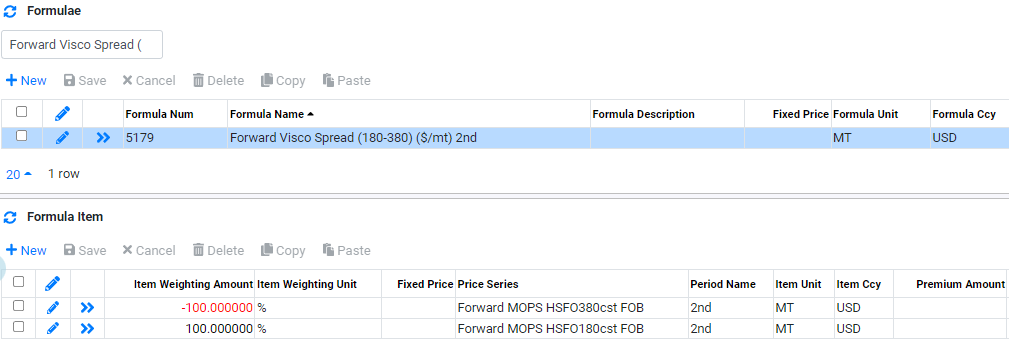For example, 7 formula is created for 7 period used for Forward Visco Spread.